## Paper Folding

#### Paper Folding

Direction: If a Paper (Transparent Sheet) is folded in a manner and a design or pattern is drawn. When unfolded this paper appears as given below in the answer figure. Choose the correct answer figure given below.

1. Find out from the four alternatives as how the pattern would appear when the transparent sheet is folded at the dotted line.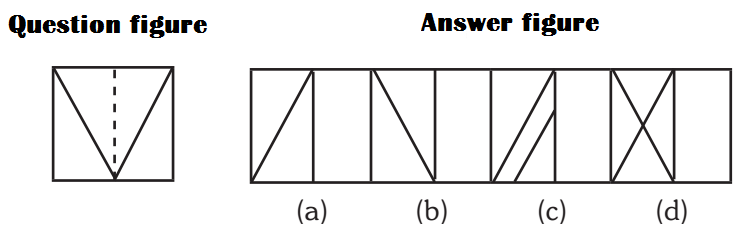1. (a)
2. (b)
3. (c)
4. (d)

1. If paper is folded from dotted line , it will make triangle.

##### Correct Option: B

Answer B

1. Find out from the four alternatives as how the pattern would appear when the transparent sheet is folded at the dotted line.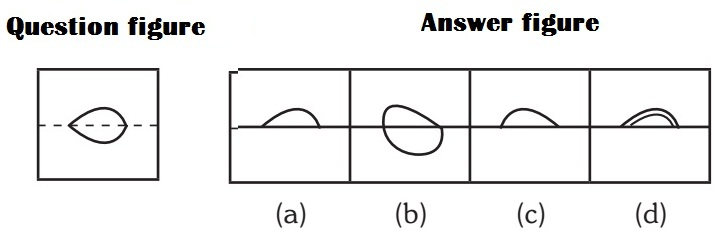1. (a)
2. (b)
3. (c)
4. (d)

1. It will cover the upper side pr lower if fold from dotted line.

##### Correct Option: A

Answer A

1. Find out from the four alternatives as how the pattern would appear when the transparent sheet is folded at the dotted line.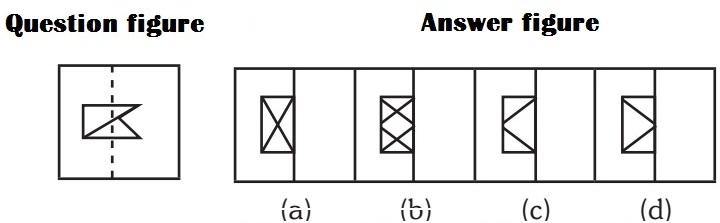1. (a)
2. (b)
3. (c)
4. (d)

1. Answer B

##### Correct Option: B

Answer B

1. Find out from the four alternatives as how the pattern would appear when the transparent sheet is folded at the dotted line.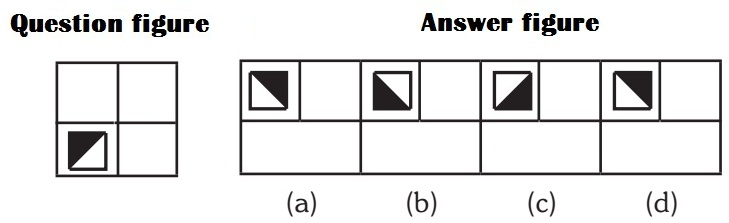1. (a)
2. (b)
3. (c)
4. (d)

1. If you fold the paper in upper side the dark area will be down with upward position.

##### Correct Option: B

Answer B

1. Find out from the four alternatives as how the pattern would appear when the transparent sheet is folded at the dotted line.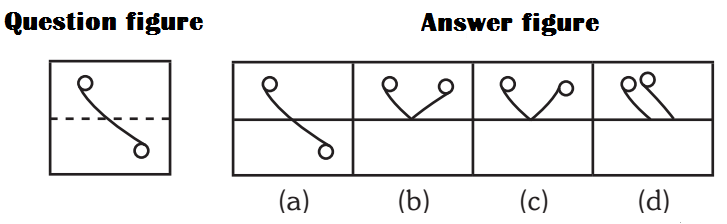1. (a)
2. (b)
3. (c)
4. (d)

1. If paper will fold from dotted line it will move the lower part in upper side.

Answer B